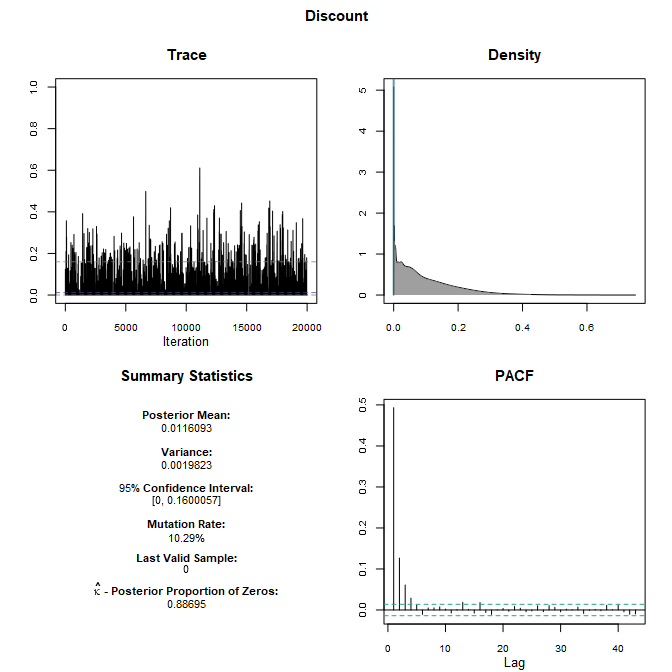# IMIFA: Infinite Mixtures of Infinite Factor Analysers and Related Models

## Introduction

IMIFA is an R package that provides flexible and efficient functions for fitting Infinite Mixtures of Infinite Factor Analysers (IMIFA) and related models. The main model, IMIFA itself, conducts Bayesian nonparametric clustering via latent Gaussian models. While the package offers a Bayesian implementation of the Factor Analysis (FA) and Mixtures of Factor Analysers (MFA) models, among others, these require pre-specification of the number of latent factors &/or the number of components, which must remain fixed, the main advantages of the IMIFA model are that a) the model search is dramatically reduced, b) these quantities are estimated automatically, c) the number of latent factors is allowed to be cluster-specific, and d) uncertainty in the number of clusters and numbers of cluster-specific factors can be quantified.

Typically, one would run FA or MFA models over ranges of values for the numbers of clusters and factors, with the pair which optimises some model selection criterion typically chosen. IMIFA instead enables Bayesian nonparametric model-based clustering with factor analytic covariance structures, without recourse to model selection criteria, to automatically choose the number of clusters &/or cluster-specific latent factors.

The main features of the IMIFA model are the multiplicative gamma process shrinkage prior on the factor loadings, which allows theoretically infinitely many factors (this can also be employed in a MIFA context, for instance, where the number of clusters is fixed but cluster-specific factors are estimated), an adaptive Gibbs sampler which dynamically truncates the infinite loadings matrices, and the Dirichlet process prior, which utilises the stick-breaking construction and slice-efficient sampling, and allows theoretically infinitely many clusters. Tools are provided for soliciting sensible hyperparameters for these priors. As of IMIFA v1.2.0, a Pitman-Yor process prior on the number of mixture components is assumed by default, and its concentration and discount parameters are learned via Metropolis-Hastings updates.

Model-specific diagnostic tools are also provided, as well as many extensive options for plotting results, conducting posterior inference on parameters of interest, and quantifying uncertainty. The functions are typically verbose, offering plenty of messages and warnings to the user where appropriate. Please see Murphy et al. (2020) for more info.

If you find bugs or want to suggest new features please visit the IMIFA GitHub issues page.

This vignette aims to reproduce some results in the Murphy et al. (2020) paper using the mcmc_IMIFA() and get_IMIFA_results() functions and demonstrates how the plots therein were created using the dedicated S3 plot method, while also demonstrating how to fit other models in the IMIFA family.

### Installing IMIFA

IMIFA will run in Windows, Mac OS X, or Linux. To install IMIFA you first need to install R. Installing RStudio as a nice desktop environment for using R is also recommended.

Once in R you can type:

install.packages('devtools')
devtools::install_github('Keefe-Murphy/IMIFA')

at the R command prompt to install the latest development version of the package from the IMIFA GitHub page.

To instead install the latest stable official release of the package from CRAN go to R and type:

install.packages('IMIFA')

In either case, if you then type:

library(IMIFA)

it will load in all the IMIFA functions.

The GitHub version contains a few more features but some of these may not yet be fully tested, and occasionally this version might be liable to break when it is in the process of being updated.

### The three main functions

There exist several utility functions in the package to solicit good prior hyperparameters (e.g. G_priorDensity(), psi_hyper(), MGP_check()) and to prepare results for producing nice plots (e.g. mat2cols()), this vignette focuses only on the three most important functions:

1.  mcmc_IMIFA()
2.  get_IMIFA_results()
3.  and a dedicated S3 plot() method for objects of class "Results_IMIFA"

While it is possible to simulate data from a factor analytic mixture using the sim_IMIFA_data() function, specifying, among other things, the sample size N, the number of clusters G, and the number of variables P, with true parameters either supplied or also simulated, e.g.

# Simulate 100 observations from 3 balanced clusters with cluster-specific numbers of latent factors
sim_data <- sim_IMIFA_data(N=100, G=3, P=20, Q=c(2, 2, 5),
psi=matrix(rgamma(60, 2, 1), nrow=20, ncol=3),
mu=matrix(rnorm(60, -2 + 1:3, 1), nrow=20, ncol=3, byrow=TRUE))

the well-known Italian olive oil data set will be used throughout this vignette instead. You can load this data set after loading the IMIFA package by typing

data(olive)

?olive

## Fitting the model & running the MCMC chain

The mcmc_IMIFA function provides an adaptive Gibbs/Metropolis-within-Gibbs sampler for nonparametric model-based clustering using models from the IMIFA family. The function facilitates model-based clustering with dimensionally reduced factor-analytic covariance structures, with automatic estimation of the number of clusters and cluster-specific factors as appropriate to the method employed. Factor analysis with one group (FA/IFA), finite mixtures (MFA/MIFA), overfitted mixtures (OMFA/OMIFA), infinite factor models which employ the multiplicative gamma process (MGP) shrinkage prior (IFA/MIFA/OMIFA/IMIFA), and infinite mixtures which employ Pitman-Yor / Dirichlet Process Mixture Models (IMFA/IMIFA) are all provided. The function creates a raw object of class 'IMIFA' from which the optimal/modal model can be extracted by get_IMIFA_results.

There are many, many options for specifying hyperparameters, specifying running conditions and pre-processing the data. These are documented both within mcmc_IMIFA and within various control functions (mixfaControl, mgpControl, bnpControl, etc.), are deferred, for brevity, to these functions’ help files. Great care was taken to ensure the default function arguments governed by the control functions would be appropriate in most applications, but you can nevertheless access further helpful instructions by typing

?mcmc_IMIFA

and ?mixfaControl, ?mgpControl, ?bnpControl etc. as needed. Arguments to these control functions can actually be supplied, provided they are named, directly to mcmc_IMIFA, and this convention is adopted throughout this document.

Be warned that the mcmc_IMIFA function calls in this section may take quite some time to run. Let’s begin by fitting a Mixture of Factor Analysers model (MFA) to the unit-scaled olive data. For this, we must specify sequences of values for range.G, the number of clusters, and range.Q, the number of latent factors. Let’s assume that uniquenesses are isotropic rather than unconstrained. This isotropic constraint provides the link between factor analysis and the probabilistic principal component analysis model (PPCA): note that we could also constrain uniqueness across clusters (but still be diagonal within each cluster) by specifying uni.type="constrained" or constrain uniquenesses to a single value (i.e. equal across all clusters and all variables) by specifying uni.type="single". Let’s elect not to store the latent factor scores, as this can be a huge drain on memory, with the caveat that posterior inference on the scores won’t be possible. Let’s also allow diagonal covariance as a special case where range.Q is 0, and accept all other defaults (for instance, cluster labels will be initialised by mclust).

simMFA   <- mcmc_IMIFA(olive, method="MFA", n.iters=10000, range.G=3:6, range.Q=0:3, centering=FALSE,
scaling="unit", uni.type="isotropic", score.switch=FALSE)

Now let’s instead have the numbers of cluster-specific latent factors be estimated automatically using a Mixture of Infinite Factor Analysers model (MIFA). This time, we’ll also mean-centre the data and initialise the cluster labels using kmeans instead. Note that range.Q no longer needs to be specified, but it can be given as a conservatively high starting value and upper limit. Let’s accept the default, and also include the Infinite Factor Analysis model (IFA) as a special case where range.G is 1.

simMIFA  <- mcmc_IMIFA(olive, method="MIFA", n.iters=10000, centering=TRUE,
range.G=1:3, z.init="kmeans")

MIFA doesn’t entirely solve the issue of model choice; as you can see, range.G still needs to be specified. We can allow the number of clusters to instead/also be estimated automatically by fitting one of the overfitted mixture models (OMFA/OMIFA) or one of the infinite mixture models (IMFA/IMIFA). Let’s fit an Overfitted Mixture of Infinite Factor Analysers, overriding the default value for the starting value / upper limit for the number of clusters (range.G), and allowing the Dirichlet hyperparameter (alpha) for the cluster mixing proportions to be learned via learn.alpha=TRUE (the default). We can enforce additional shrinkage by varying other MGP hyperparameters, using arguments from mgpControl().

simOMIFA <- mcmc_IMIFA(olive, method="OMIFA", n.iters=10000, range.G=10, learn.alpha=TRUE,
nu=3, alpha.d1=3.5, alpha.d2=7, prop=0.8, epsilon=0.01)

Finally, let’s run the flagship IMIFA model, on which all subsequent demonstrations and results will be based, for a greater number of iterations, accepting the defaults for most arguments. Note that the verbose argument, which defaults to TRUE will ordinarily print a progress bar to the console window. The default implementation uses the independent rather than dependent slice-efficient sampler; we could override the default for the parameter governing the rate of geometric decay by specifying rho.

simIMIFA <- mcmc_IMIFA(olive, method="IMIFA", n.iters=50000, verbose=FALSE)

## Postprocessing and extracting optimum results

In order to extract results, conduct posterior inference and compute performance metrics for MCMC samples of models from the IMIFA family, we can pass the output of mcmc_IMIFA to the function get_IMIFA_results(). If, for instance, simMFA above was supplied, this function would find the pair of $$G$$ and $$Q$$ values which optimises a model selection criterion of our choosing and prepare results from that model only. If simIMIFA is supplied, this function finds the modal estimates of $$G$$ and each $$q_g$$ (the cluster-specific number of latent factors), and likewise prepares results accordingly.

This function can be re-ran at little computational cost in order to extract different models explored by the sampler used by mcmc_IMIFA, without having to re-run the model itself. New results objects using different numbers of clusters and different numbers of factors (if visited by the model in question), or using different model selection criteria (if necessary) can be generated with ease. The function also performs post-hoc corrections for label switching, as well as post-hoc Procrustes rotation to ensure sensible posterior parameter estimates, computes error metrics, and constructs credible intervals, the average similarity matrix, and the posterior confusion matrix. Please see the function’s help manual by typing ?get_IMIFA_results for further assistance with the various function arguments.

If we wanted to choose the optimum MFA model, we would simply type

resMFA  <- get_IMIFA_results(simMFA)

If we instead wanted to explore the 3-cluster solution and have the number of latent factors chosen by another criterion, we could try

resMFA2 <- get_IMIFA_results(simMFA, G=3, criterion="aic.mcmc")

For now, let’s just extract results from our IMIFA run above so we can proceed to visually examine them. Though the IMIFA model obviates the need for model selection criteria, the syntax for extracting results is exactly the same. However, this time, let’s also summarise the clustering via the $$N * N$$ similarity matrix obtained by averaging the adjacency matrices (admittedly at the expense of slightly slowing the function down!), so that we can visualise it later.

resIMIFA <- get_IMIFA_results(simIMIFA, z.avgsim=TRUE)

Before we examine the results in great detail, we can quickly summarise the solution as follows

summary(resIMIFA, MAP=TRUE)
#> Call:    get_IMIFA_results.IMIFA(sims = simIMIFA, z.avgsim = TRUE)
#>
#> The chosen IMIFA model has 4 groups with 6, 3, 6 and 2 factors respectively:
#> this Results_IMIFA object can be passed to plot(...)
#>
#> Clustering table :
#>   1   2   3   4
#> 323  98 103  48

## Visualing IMIFA results

The object resIMIFA above is of the class Results_IMIFA. We can call plot on objects of this class to access a dedicated function for visualising output and parameters of inferential interest for IMIFA and related models. The two most important arguments, beyond the Results_IMIFA object itself, are plot.meth and param, where the former dictates the type of plot to be produced (one of c("all", "correlation", "density", "errors", "GQ", "means", "parallel.coords", "trace", "zlabels"), depending on the method employed originally by mcmc_IMIFA) for the parameter of interest (one of c("means", "scores", "loadings", "uniquenesses", "pis", "alpha"), depending on the method employed originally by mcmc_IMIFA). Note that "all" refers here to the options "trace", "density", "means", and "correlation". Note also that many of the function calls below will also print relevant output to the console window that is not always shown here. Please see the function’s help manual by typing plot.Results_IMIFA for further assistance with the various function arguments.

Let’s examine the posterior distribution of $$G$$ and the posterior distribution of $$q_g$$ for each of the 4 clusters. The third plot below, depicting the trace of the numbers of active and non-empty clusters, allows us to examine mixing of the chain with respect to $$G$$. The true number of clusters is estimated by $$G=4$$, the modal value.

plot(resIMIFA, plot.meth="GQ")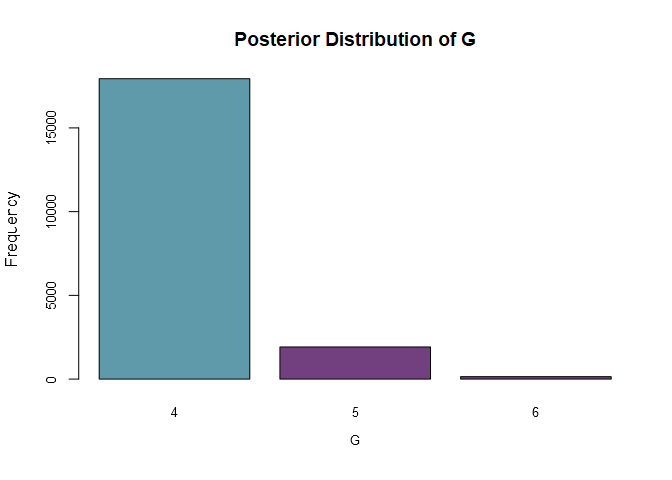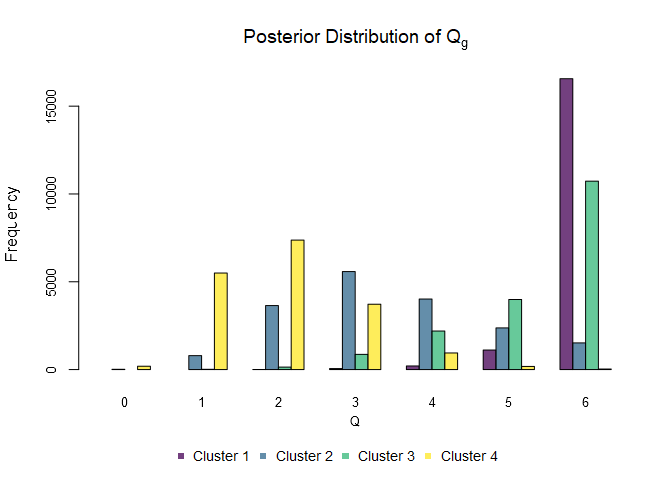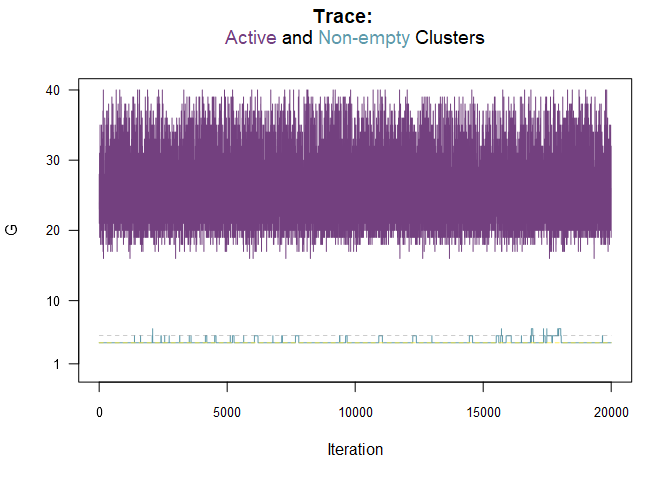Let’s examine clustering performance against the known cluster labels. Note that the cluster labels could have been already supplied to get_IMIFA_results too, but plotting allows clustering performance to be evaluated against new labels without having to extract the full results object all over again. More specifically, the code below allows us to visualise the clustering uncertainty (with or without the labels being supplied; in fact, when they are supplied, misclassified observations are highlighted, otherwise observations with uncertainty exceeding the inverse of the number of clusters are highlighted).

plot(resIMIFA, plot.meth="zlabels", zlabels=olive$area, g=1) #> confusion.matrix : #> Observed #> Predicted Southern Italy Sardinia Northern Italy Sum #> 1 323 0 0 323 #> 2 0 98 0 98 #> 3 0 0 103 103 #> 4 0 0 48 48 #> Sum 323 98 151 572 #> #> rand : #>  0.9697255 #> #> crand : #>  0.9370795 #> #> misclassified : #>  422 423 424 425 426 427 428 429 430 431 432 433 434 435 436 437 438 439 440 #>  441 442 443 444 445 446 447 448 449 450 451 452 453 454 455 456 457 458 459 #>  461 462 463 464 465 467 469 470 471 472 #> #> error.rate : #>  "8.39%"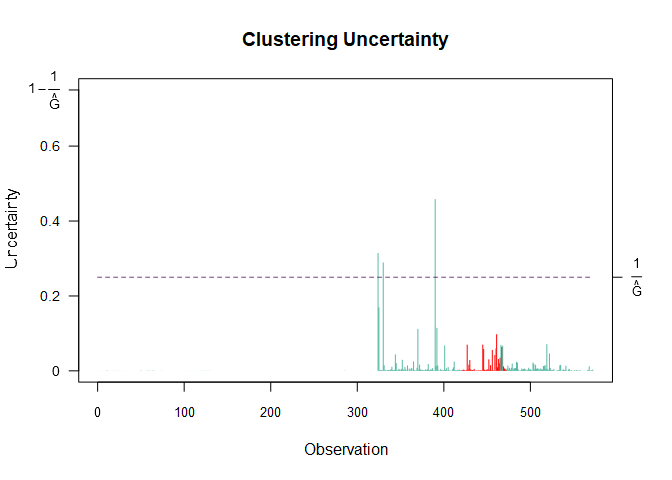We can also plot a clustering uncertainty profile, also highlighting misclassified observations, with g=2. When plot.meth="zlabels", g=3 would mean to instead visualise the uncertainties in the form of a histogram. plot(resIMIFA, plot.meth="zlabels", zlabels=olive$area, g=2)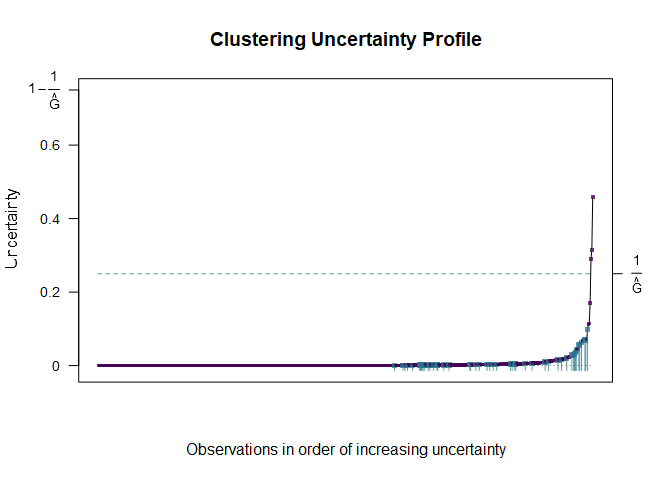With g=4, we can visualise the posterior confusion matrix. The benchmark matrix for comparison is the identity matrix of order $$G=4$$, corresponding to a situation with no uncertainty in the clustering.

plot(resIMIFA, plot.meth="zlabels", g=4)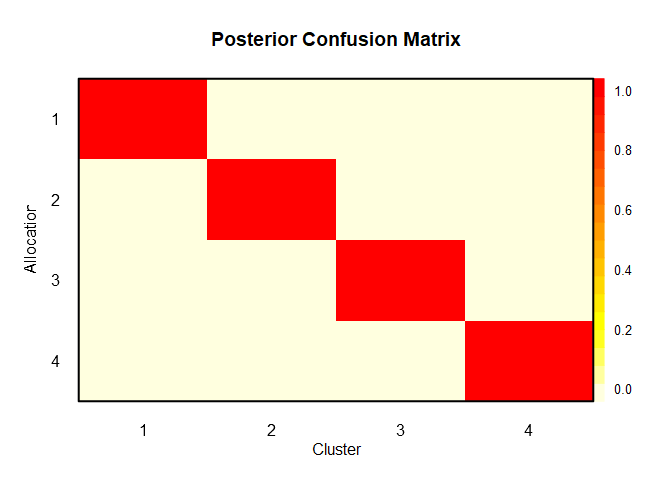Finally, we can visualise the $$N * N$$ similarity matrix by supplying g=5 when plot.meth="zlabels". Had we not specified g, the function would cycle through the available plots.

plot(resIMIFA, plot.meth="zlabels", g=5)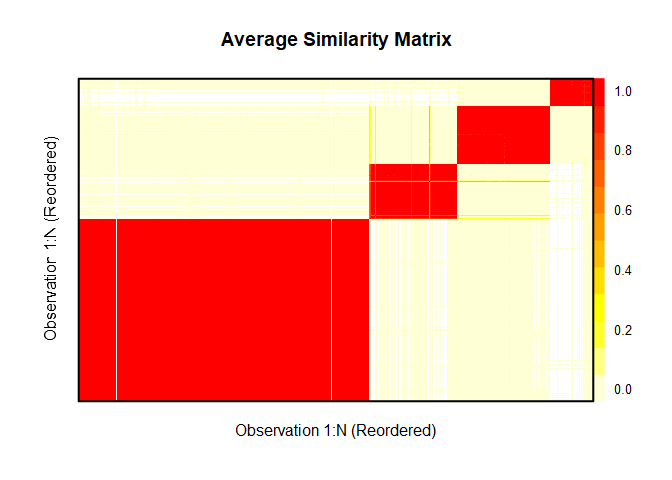To examine the posterior mean estimates of the cluster means in a given cluster (say the 1st), we can set both plot.meth and param to "means". If the cluster isn’t specified using the argument g, the user will be prompted to hit <Return> at the onset of each plot in order to cycle through similar plots for all clusters. In the code below mat=TRUE means, in this case, to plot all variables simultaneously. By default, credible intervals are also plotted. Note that the data were originally mean-centred and unit-scaled when mcmc_IMIFA was called.

plot(resIMIFA, plot.meth="means", param="means", mat=TRUE, g=1)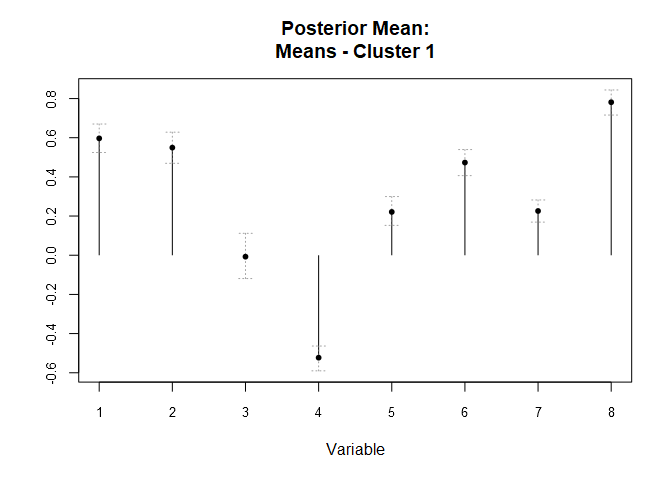Had the factor scores been stored, we could examine the trace plots for them using the code below, where mat=TRUE and by.fac=FALSE (the default) means, in this case, to plot all factors simultaneously for a given observation: ind=1 specifies that the observation of interest is the first (however this is not shown, as the scores were not stored).

plot(resIMIFA, plot.meth="trace", param="scores", mat=TRUE, ind=1)

We could instead plot all observations simultaneously for a given factor, say the 2nd (also not shown).

plot(resIMIFA, plot.meth="trace", param="scores", mat=TRUE, by.fac=TRUE, fac=2)

The code below will produce only a heat map of the loadings matrix in the first cluster: note, however, that heat.map=TRUE by default for loadings (whereas the opposite is true for the scores). Darker colours correspond to entries which are more negatively loaded and vice versa.

plot(resIMIFA, plot.meth="means", param="loadings", heat.map=TRUE, g=1)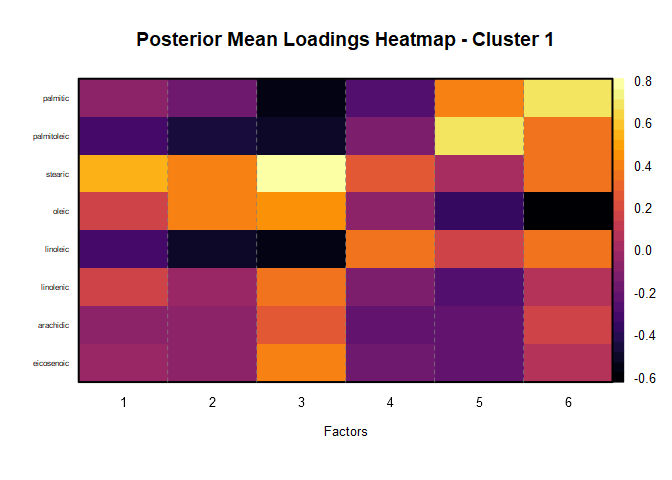To examine posterior mean uniquenesses from all clusters in the form of a parallel coordinates plot, type

plot(resIMIFA, plot.meth="parallel.coords", param="uniquenesses")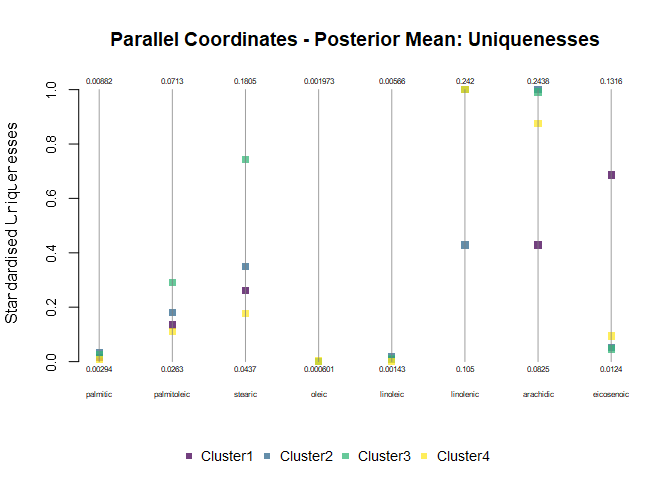We could have also used the argument show.last to visualise the last valid sample of uniquenesses from all clusters instead. This argument can be used to replace the posterior mean by the corresponding last valid sample for any combination of arguments to plot.Results_IMIFA that shows a posterior mean.

Posterior predictive checking to assess the appropriateness of the fitted model is also facilitated. The posterior predictive reconstruction error, obtained by comparing bin counts of the data against bin counts of replicate draws from the posterior distribution, can be visualised as follows. Setting g=2 allows individual histograms to be depicted, while the PPRE offers an overall perspective across variables.

plot(resIMIFA, plot.meth="errors", g=1)
#>              2.5%              Mean            Median Last Valid Sample
#>        0.09039207        0.10659232        0.10605268        0.10396050
#>             97.5%
#>        0.12578787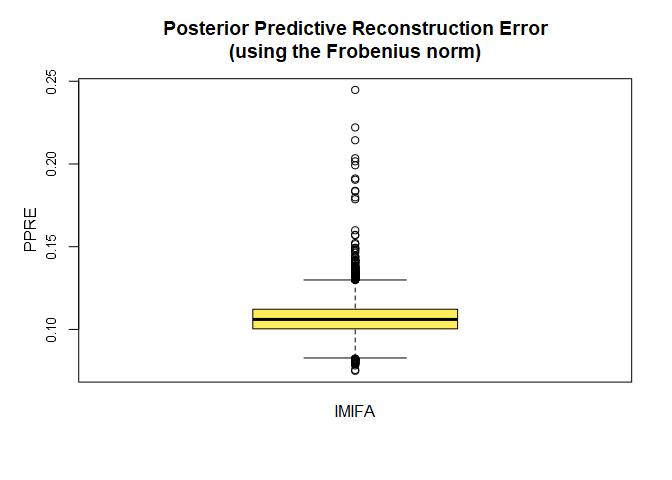Quantifying the error between the empirical and estimated covariance matrices - and the uncertainty associated with those metrics - can also provide a useful indicator of the validity of the solution. For models which achieve clustering, the overall estimated covariance matrix is constructed from the cluster-specific estimated covariance matrices. A visualisation can be produced as follows:

plot(resIMIFA, plot.meth="errors", g=3)
#>                                        MSE        MEDSE        MAE      MEDAE
#> Medians                        0.003668130 0.0014637191 0.04738250 0.03824102
#> Evaluated at Posterior Mean    0.001107329 0.0001106886 0.02280001 0.01052086
#> Evaluated at Last Valid Sample 0.002688072 0.0016590855 0.04191812 0.04073185
#>                                      RMSE      NRMSE
#> Medians                        0.06056509 0.03269479
#> Evaluated at Posterior Mean    0.03327655 0.01796365
#> Evaluated at Last Valid Sample 0.05184662 0.02798831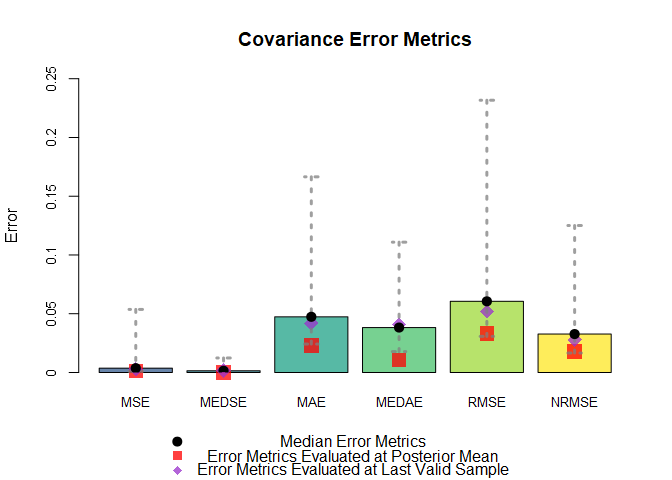Finally, inference can be conducted on the Pitman-Yor concentration parameter $$\alpha$$ and discount parameter $$d$$ (assuming learn.alpha and learn.d, respectively, were not set to FALSE when mcmc_IMIFA was initially run using the IMFA/IMIFA methods), where all below refers to trace, density, posterior means, and ACF/PACF (correlation) plots. The type of correlation plot can be toggled via the logical argument partial. Note that the density for discount accounts for the point-mass at zero built into its prior.

plot(resIMIFA, plot.meth="all", param="alpha")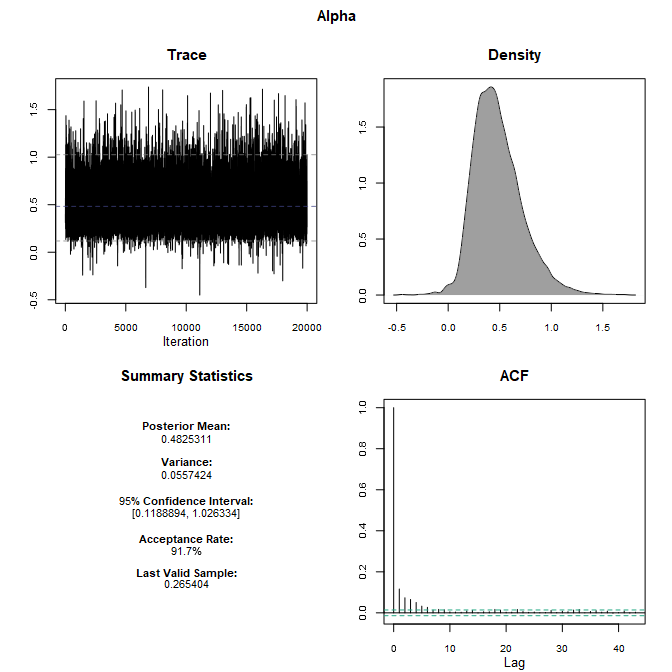plot(resIMIFA, plot.meth="all", param="discount", partial=TRUE)